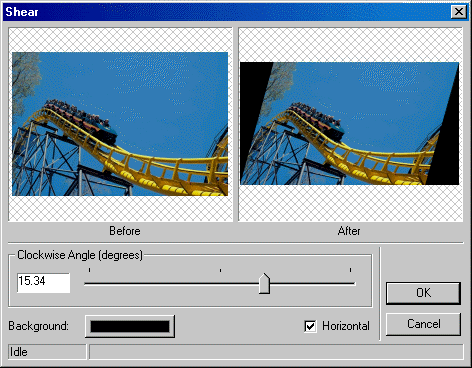←Select platform
In This Topic ▼

# ShearCommand Class

Summary
Moves the corners of an image in the fashion of a parallelogram.
Syntax
C#
VB
Objective-C
C++
Java
``public class ShearCommand : RasterCommand ``
````Public Class ShearCommand  `
`   Inherits RasterCommand ````
``@interface LTShearCommand : LTRasterCommand ``
``public class ShearCommand extends RasterCommand ``
``public ref class ShearCommand : public RasterCommand   ``
Remarks

You specify an angle of slant, horizontal or vertical shear, and the fill color for the created space. The following is a horizontal example:The angle is expressed in hundredths of degrees, with a limit of 4500 (45 degrees).

This command supports signed/unsigned data images.

Example

This example shears the image 15 degrees vertically, filling extra space with blue.

C#
VB
````using Leadtools; `
`using Leadtools.Codecs; `
`using Leadtools.ImageProcessing; `
` `
` `
`public void ShearCommandExample() `
`{ `
`	RasterCodecs codecs = new RasterCodecs(); `
` `
`	string srcFileName = Path.Combine(LEAD_VARS.ImagesDir, "Image1.cmp"); `
`	string destFileName = Path.Combine(LEAD_VARS.ImagesDir, "ShearCommand.bmp"); `
` `
`	// Load the source image from disk `
`	RasterImage image = codecs.Load(srcFileName); `
` `
`	// Shear the image 15 degrees vertically `
`	ShearCommand command = new ShearCommand(); `
`	command.Angle = -15 * 100; `
`	command.FillColor = new RasterColor(255, 255, 255); `
`	command.Horizontal = false; `
`	command.Run(image); `
` `
`	// Save the image back to disk `
`	codecs.Save(image, destFileName, RasterImageFormat.Bmp, 32); `
` `
`	// Clean Up `
`	image.Dispose(); `
`	codecs.Dispose(); `
`} `
` `
`static class LEAD_VARS `
`{ `
`   public const string ImagesDir = @"C:\LEADTOOLS22\Resources\Images"; `
`} ````
````Imports Leadtools `
`Imports Leadtools.Codecs `
`Imports Leadtools.ImageProcessing `
` `
`Public Sub ShearCommandExample() `
`   Dim codecs As RasterCodecs = New RasterCodecs() `
` `
`   Dim srcFileName As String = Path.Combine(LEAD_VARS.ImagesDir, "Image1.cmp") `
`   Dim destFileName As String = Path.Combine(LEAD_VARS.ImagesDir, "ShearCommand.bmp") `
` `
`   ' Load the source image from disk `
`   Dim image As RasterImage = codecs.Load(srcFileName) `
` `
`   ' Shear the image 15 degrees vertically `
`   Dim command As ShearCommand = New ShearCommand() `
`   command.Angle = -15 * 100 `
`   command.FillColor = New RasterColor(255, 255, 255) `
`   command.Horizontal = False `
`   command.Run(image) `
` `
`   ' Save the image back to disk `
`   codecs.Save(image, destFileName, RasterImageFormat.Bmp, 32) `
` `
`   ' Clean Up `
`   image.Dispose() `
`End Sub `
` `
`Public NotInheritable Class LEAD_VARS `
`   Public Const ImagesDir As String = "C:\LEADTOOLS22\Resources\Images" `
`End Class ````
Requirements